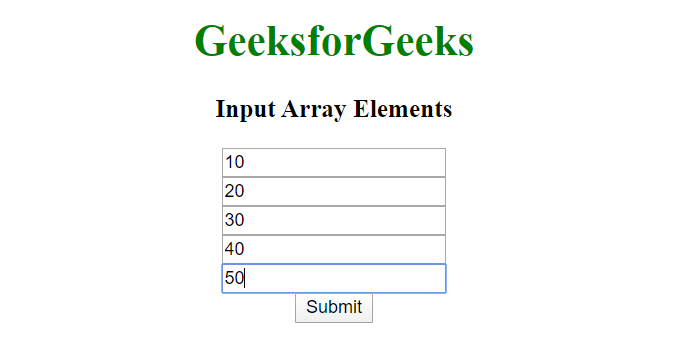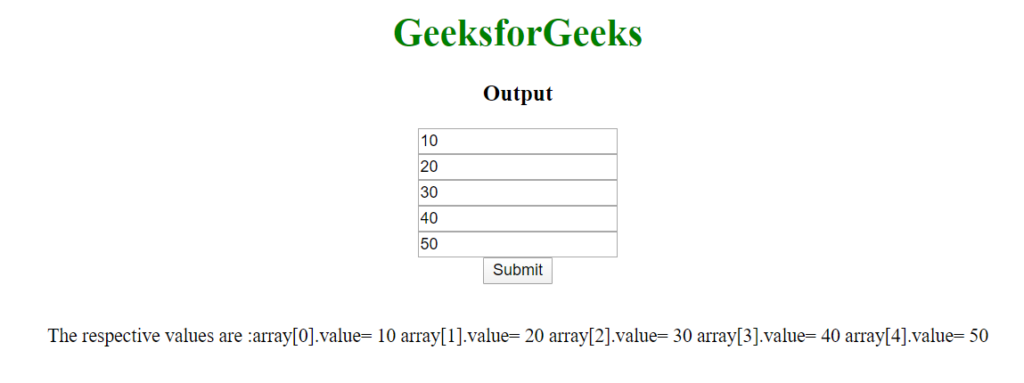# How to get values from html input array using JavaScript ?

This problem can be solved by using input tags having the same “name” attribute value that can group multiple values stored under one name which could later be accessed by using that name. To access all the values entered in input fields use the following method:

`var input = document.getElementsByName('array[]');`

The document.getElementsByName() method is used to return all the values stored under a particular name and thus making input variable an array indexed from 0 to number of inputs. The method document.getElementById().innerHTML is used to change the inner HTML of selected Id. So in this way, we can access the input values and convert them into the array and access it as you like. We have used form tag because it is generally a good practice to group all the input elements together inside a form otherwise in the present scenario it is completely optional.

Example:

 ` ` `<``html` `lang``=``"en"` `dir``=``"ltr"``> ` ` `  `<``head``> ` `    ``<``meta` `charset``=``"utf-8"``> ` `    ``<``title``> ` `        ``How to get values from html input ` `        ``array using JavaScript ? ` `    `` ` ` ` ` `  `<``body` `style``=``"text-align: center;"``> ` `     `  `    ``<``h1` `style``=``"color: green;"``> ` `        ``GeeksforGeeks ` `    `` ` ` `  `    ``<``h3` `id``=``"po"``>Input Array Elements ` `    ``<``form` `class``=``""` `action``=``"index.html"` `method``=``"post"``> ` `        ``<``input` `type``=``"text"` `name``=``"array[]"` `value``=``""` `/><``br``> ` `        ``<``input` `type``=``"text"` `name``=``"array[]"` `value``=``""` `/><``br``> ` `        ``<``input` `type``=``"text"` `name``=``"array[]"` `value``=``""` `/><``br``> ` `        ``<``input` `type``=``"text"` `name``=``"array[]"` `value``=``""` `/><``br``> ` `        ``<``input` `type``=``"text"` `name``=``"array[]"` `value``=``""` `/><``br``> ` `        ``<``button` `type``=``"button"` `name``=``"button"` `onclick``=``"Geeks()"``> ` `            ``Submit ` `        `` ` `    `` ` `    ``<``br``> ` ` `  `    ``<``p` `id``=``"par"``> ` ` `  `    ``<``script` `type``=``"text/javascript"``> ` `        ``var k = "The respective values are :"; ` `        ``function Geeks() { ` `            ``var input = document.getElementsByName('array[]'); ` ` `  `            ``for (var i = 0; i < ``input.length``; i++) { ` `                ``var ``a` `= ``input``[i]; ` `                ``k` `= k + "array[" + i + "].value= " ` `                                   ``+ a.value + " "; ` `            ``} ` ` `  `            ``document.getElementById("par")``.innerHTML` `= ``k``; ` `            ``document.getElementById("po")``.innerHTML` `= ``"Output"``; ` `        ``} ` `    `` ` ` ` ` `  ` `

Output:

• Input Array Elements:• Output Array Elements:My Personal Notes arrow_drop_upCheck out this Author's contributed articles.

If you like GeeksforGeeks and would like to contribute, you can also write an article using contribute.geeksforgeeks.org or mail your article to contribute@geeksforgeeks.org. See your article appearing on the GeeksforGeeks main page and help other Geeks.

Please Improve this article if you find anything incorrect by clicking on the "Improve Article" button below.

Practice Tags :

1

Please write to us at contribute@geeksforgeeks.org to report any issue with the above content.